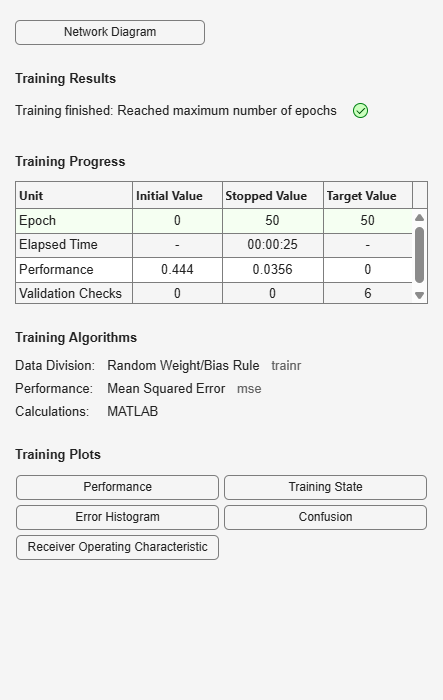Main Content

# lvqnet

Learning vector quantization neural network

## Syntax

```lvqnet(hiddenSize,lvqLR,lvqLF) ```

## Description

LVQ (learning vector quantization) neural networks consist of two layers. The first layer maps input vectors into clusters that are found by the network during training. The second layer merges groups of first layer clusters into the classes defined by the target data.

The total number of first layer clusters is determined by the number of hidden neurons. The larger the hidden layer the more clusters the first layer can learn, and the more complex mapping of input to target classes can be made. The relative number of first layer clusters assigned to each target class are determined according to the distribution of target classes at the time of network initialization. This occurs when the network is automatically configured the first time `train` is called, or manually configured with the function `configure`, or manually initialized with the function `init` is called.

`lvqnet(hiddenSize,lvqLR,lvqLF)` takes these arguments,

 `hiddenSize` Size of hidden layer (default = 10) `lvqLR` LVQ learning rate (default = 0.01) `lvqLF` LVQ learning function (default = `'learnlv1'`)

and returns an LVQ neural network.

The other option for the `lvq` learning function is `learnlv2`.

## Examples

### Train a Learning Vector Quantization Network

Here, an LVQ network is trained to classify iris flowers.

```[x,t] = iris_dataset; net = lvqnet(10); net.trainParam.epochs = 50; net = train(net,x,t); view(net) y = net(x); perf = perform(net,y,t) classes = vec2ind(y); ```
```perf = 0.0489 ```## See Also

Introduced in R2010b

Download ebook